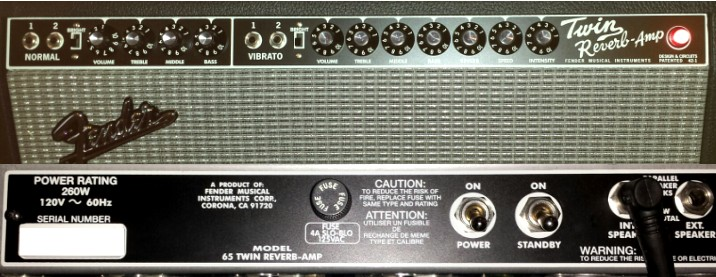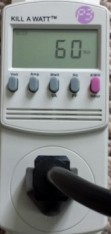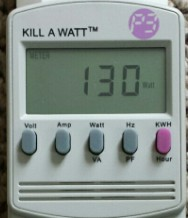'65 Twin Reverb Amp Power Measurements

June 07, 2014

This article presents line power measurements for the vintage reissue Fender '65 Twin Reverb guitar amplifier model AB 763.Proper bias adjustment of tube amplifiers can be performed accurately using a bias tool which directly measures the cathode current of each output 6L6 power tube. The power dissipation of the amplifier as a whole can also be measured using the amplifier input line power using an AC power meter such as the P4400 Kill A Watt device. The measurements below show that this type of measurement is consistent with direct power tube bias measurements.

Standby Power MeasurementsIn the amplifier STANDBY power position, heater current is applied to all amplifier tubes, but the plate voltage to all tubes is disconnected. In this position, it is easy to determine the heater power delivered to the power tubes alone by removing pairs of power tubes and comparing this power with the power with all power tubes present. Using the P4400 Kill A Watt power measuring device, the STANDBY power measurements are:
```  60 W   (4 power tubes)
47 W   (2 power tubes)
34 W   (0 power tubes)
```
Therefore 4 power tubes contribute 26W of heater dissipation, or 6.5W per power tube, consistent with the 6L6 power tube filament current and voltage..

ON Power MeasurementsIn the amplifier ON power position, heater current and plate voltage are applied to the amplifier circuit. Without an input signal, the "idle" power dissipation is 130W as shown with the power tubes biased normally to an average value of Ip ~ 32mA. Comparing to the STANDBY power, most of the extra power (130-60 = 70W) is power tube idle plate dissipation due to the bias current. However a portion of this power difference is related to applying plate power to the other tubes in the circuit. This can be easily determined by removing power tubes and again measuring the total power in the ON position:
``` 130 W   (4 power tubes)
88 W   (2 power tubes)
43 W   (0 power tubes)
```
If the power tube heater current as determined above is added to the 43W power with no power tubes, we obtain the total circuit power dissipation for zero plate dissipation or zero bias current (43+26 = 69W). The power tube plate dissipation for the normally biased power tubes is therefore 130 - 69 = 61W or 15.3W per power tube, in good agreement with the power tube plate dissipation of 14.6W determined directly from the measured cathode bias current of Ip ~ 32mA at Vbb = 455VDC. If the bias adjustment on the Twin Reverb amp is adjusted to its minimum position of Ip ~ 4mA, the total measured power is 75W which means 75-69 = 6W plate dissipation for 4 tubes, in good agreement with 7W as calculated directly from the 4mA and 455Vbb.

Maximum Audio Power Measurements

Finally, a 440 Hz audio signal was applied to the ampllifier input and the level adjusted to drive the amplifier to maximum output power (nominally 85W) into a 4Ω resistive load. In this case the total measured line power for the Twin Reverb amp was 250W (Note that the Power Rating stated on the Twin Reverb chassis is 260W.). The plate dissipation under maximum output signal swing is approximately the same as the idle power dissipation at Ip ~ 32 mA of 15W/power-tube. Therefore the power difference 250 - 130 = 120W is the power delivered to the primary of the audio output transformer. This primary output power agrees well with that calculated for the Twin Reverb circuit. Assuming an output power transformer efficiency of 70% provides good agreement with the measured 84W output power across the 4Ω load. Direct plate voltage and current measurements on the output transformer primary and simultaneous transformer secondary output power measurements into a resistive load confirm an output transformer power efficiency of ~ 70% at a frequency of 440Hz.

References: